## Sunday, 22 March 2020

### The ArduINA226 power monitor

Introduction
In the past I have developed various projects of ammeters based on Hall effect current sensors such as the ACS712, or on High-Side Current-Sense Amplifiers such as the MAX4080SASA or made with operational amplifiers. All these systems have an analog output which must then be digitized. The INA226 sensor has a digital output and incorporates a 16-bit ADC for which a high accuracy and precision is obtained.
It measures current and voltage and calculates power while Arduino communicates with the chip, presents the measurements on an LCD display and stores them on a micro SD card. This chip operates with a maximum voltage of 36 volts while the current is limited only by the shunt used.
There are some libraries for the INA226 chip, I used the Korneliusz Jarzebski library which seems to me quite complete even if I had to make some changes to two functions.
There are numerous possible applications for this monitoring tool: battery-powered devices such as scooters or pedal-assisted bicycles, photovoltaic panels, etc.

The INA226 sensor
In current measurements with the shunt there are two ways to insert it:
1)      To ground (low-side): the shunt is connected between the load and the ground.
2)      Towards the power supply (high-side): the shunt is connected between the power supply and the load.
The INA226 integrated circuit, by Texas Instruments, is a digital device that measures the current with a high-side or low-side shunt and also measures the voltage, calculates the power and provides a multifunctional alarm. The functional scheme is visible in figure 1.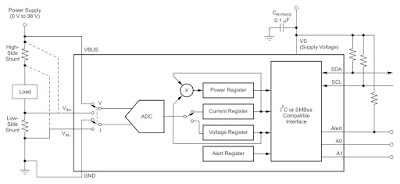Figure 1

The resolution of the shunt voltage is 2.5 mV with a full scale of 32768x2.5 = 81.92mV. For the VBUS voltage the resolution is 1.25 mV with a theoretical full-scale of 40.96 V even if the 36 V must not be exceeded. The resolution of the power is 25 times that of the current, with a full-scale that depends on the shunt used. So the system has a remarkable measurement accuracy.
The internal ADC is based on a 16-bit delta-sigma converter (ΔΣ) with a typical sampling frequency of 500 kHz (± 30%), so it is also suitable for currents that change rapidly over time.
The chip has 10 pins and very small dimensions, with a DGS case (VSSOP).
The INA226 is able to provide a hardware or software alert if a variable, selected by the user, has exceeded a limit. The user can select one of the five functions available to monitor and/or set the Conversion Ready bit. The five alert functions that can be monitored are:
Shunt Voltage Over-Limit (SOL): exceeding the maximum current threshold;
Shunt Voltage Under-Limit (SUL): exceeding the minimum current threshold;
Bus Voltage Over-Limit (BOL): exceeding the maximum voltage threshold;
Bus Voltage Under-Limit (BUL): exceeding the minimum voltage threshold;
Power Over-Limit (POL): exceeding the maximum power threshold;
The Alert output is open-drain and can be easily connected to a blocking device. The Alert output is open-drain and can be easily connected to a blocking circuit. For simplicity, I preferred to read the Shunt Voltage Over-Limit (SOL) type alert via software and present it on the display.

The INA226 module
On the market there are small breakout boards such as the one I used, visible in figure 2, of which I obtained the diagram in figure 3.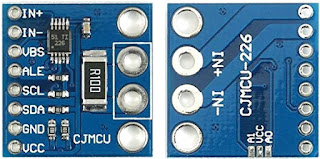Figure 2Figure 3
The device has two address pins, A0 and A1. The following table lists the pin connections for each of the 16 possible addresses.

 A1 A0 Slave Address A1 A0 Slave Address GND GND 1000000 SDA GND 1001000 GND VS 1000001 SDA VS 1001001 GND SDA 1000010 SDA SDA 1001010 GND SCL 1000011 SDA SCL 1001011 VS GND 1000100 SCL GND 1001100 VS VS 1000101 SCL VS 1001101 VS SDA 1000110 SCL SDA 1001110 VS SCL 1000111 SCL SCL 1001111
The module mounts two pull-down resistors (R2 and R3), therefore the address is 0x40 if we don’t connect the jumpers placed on the opposite side to that of the components (see figure 2 on the right).
With the shunt resistor of 0.1 W (R100), mounted on the module, there is a current resolution of 2.5 mV / 0.1 = 0.025 mA, a full scale of 81.92mV/0.1W = 819.2 mA and a power resolution of 0.025 mA *25 = 0.625 mW. I preferred to mount an external shunt for high currents, unsolder the internal one, and insert an RC filter, as shown in the diagram in figure 6.

The LCD display
I used a common two-line 16-character LCD display with Hitachi HD44780 compatible controller and a backlit equipped with high efficiency LED diodes that consumed about 20 mA, which I reduced to 10 mA with an external resistor in series with the internal one. The connections with Arduino Nano are as follows:
 LCD pin function Arduino  pin LCD pin function Arduino  pin 14 D7  Data Bus Line A3 5 R/W - Read/Write Gnd 13 D6  Data Bus Line A2 4 RS - Register Select D7 12 D5  Data Bus Line D4 3 VEE - 11 D4  Data Bus Line D5 2 VCC (+5V) VCC 6 E - Enable Signal D6 1 VSS (GND) Gnd

The micro SD module
In the latest version I added an SD module suitable for Arduino, that is, with 5 V power supply and logic levels. The appearance of the module used is visible in figure 4.Figure 4
The following table shows the connections and pins dedicated to the microSD:
 J2 pin SD module name Arduino pin 6 CS D10 5 SCK D13 4 MOSI D11 3 MISO D12 2 VCC +5 V 1 GND GND

Calculations for the shunt used
I used a shunt I had at home, visible in figure 5, it was an accessory of the glorious ICE 680R analog tester.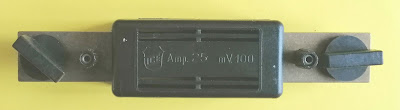Figure 5
As can be seen from the figure, it has an output of 100 mV with a current of 25 A, so Rs = 0.1/25 = 0.004 Ω.

I unsoldered the 0.1 Ω shunt, mounted on the INA226 module, and I soldered a 1 MF capacitor and two filter resistors. This expedient allows RC filtering, as suggested by the chip manufacturer.
With the full-scale shunt voltage in mind, the maximum measurable current now becomes:
Ifs = 81.92mV/4 mΩ = 20.48 A
The current resolution will be: 20.48 / 32768 = 0.625 mA.
The calibrate function has as input parameters the shunt resistance and the maximum current, in our case: rShunt = 0.004 Ω and iMaxExpected = 20.48 A.
It calculates several variables:
currentLSB = iMaxExpected / 32768 = 20.48 / 32768 = 0.625 [mA]
calibrationValue = 0.00512 / currentLSB / rShunt = 0.00512 / 0.000625 / 0.004 = 2048
powerLSB = currentLSB * 25 = 0.000625 * 25 = 15.625 [mW]
Another data to pass to the program is the current threshold of the setShuntVoltageLimit function.
If we set the upper limit of the instrument at 20 A, we can calculate:
setShuntVoltageLimit = 0.004 Ω * 20 A = 0.08 V

The prototipe
I used an Arduino Nano, of course you can use other Arduino boards, such as Arduino Uno or Arduino Pro but this board is very compact, complete with USB adapter, and can be mounted on a prototype pre-drilled pcb.
Arduino Nano has its own 5V regulator, a Low DropOut type AMS1117, but I preferred to power the system with a common LM7805, this is because the former accepts a maximum input voltage of 15V against the 35V of the second. If I have to power ArduINA226 with the voltage I have to monitor, it is better to have compatible values. Another reason is the backlight of the display. The consumption of the whole system is around 45 mA. The Schottky D1 diode, which has a drop of only 0.2V, is used to avoid conflicts between external power supply and USB power supply.
Figure 6 shows the wiring diagram of my realization.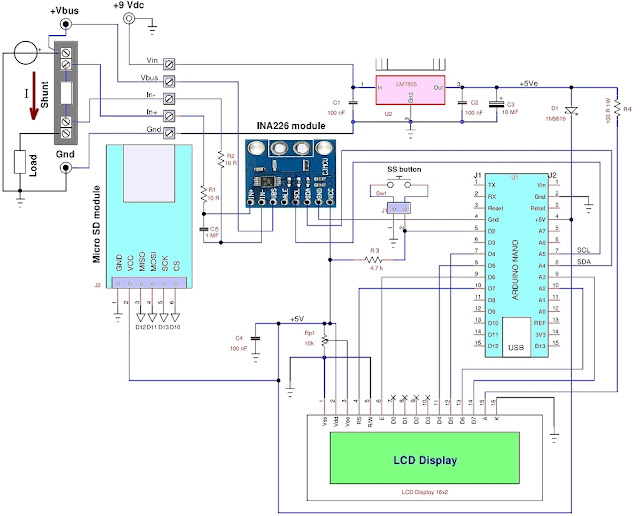Figure 6

List of components used
 component description component description C1,C2,C4 100 nF 50V, ceramic capacitor R4 100 ohm, ±5%, 0.5 W resistor C3 10 MF 50V, electrolytic capacitor U1 Arduino Nano board C4 1 MF 25V, ceramic capacitor U2 LM7805, 5V regulator D1 1N5819, Schottky diode U3 INA226 module Rp1 22kW resistive trimmer display 2 rows x 16 columns LCD R1, R2 10 ohm, ±5%, 0.25 W resistor modulo SD micro SD board with 5V levels R3 4.7 kohm, ±5%, 0.25 W resistor Sw1 Normally Open (NO) push button
Note: capacity prefix 'M' stands for microfarad (1e-6 F)
Figures 7 shows the appearance of my prototype. I screwed the shunt directly on the instrument case, note also the slot for the micro SD card.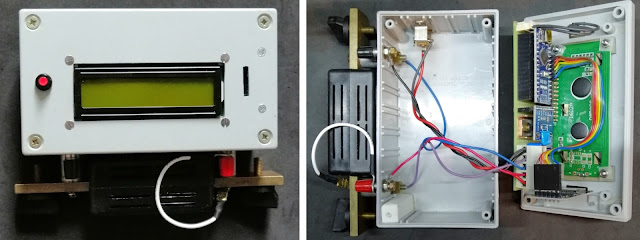Figure 7
The tests
Figure 8 shows the instrument running during the tests.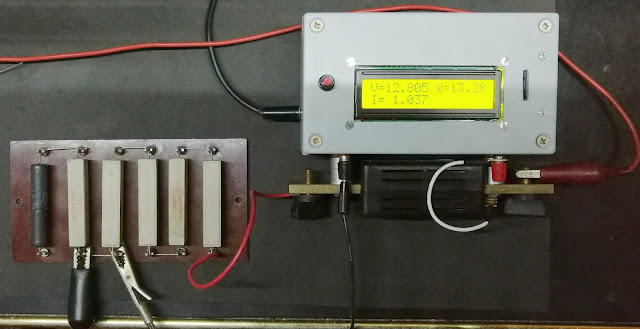Figure 8
With a ten measuring points, compared with a precision multimeter, the results have been very good, as can be seen from the graph in figure 9.
In my case, the VBUS voltage was very accurate and required no correction. The current is slightly lower than the expected value (correction of 1.0092) and the linearity was excellent with R = 0.999995.

Changes to the INA226.cpp library
There are some libraries for the INA226 chip, I used the Korneliusz Jarzebski library which seems to me quite complete. I changed the calibrate function because the shunt current was incorrect. The setShuntVoltageLimit function was also wrong. Then I realized that the latter, in version 1.1 of the library, had been corrected. Here are the modified functions, to replace the original ones:
bool INA226::calibrate(float rShunt, float iMaxExpected){ // MODIFIED by GCAR
uint16_t calibrationValue;
float iMaxPossible;
iMaxPossible = vShuntMax / rShunt;
currentLSB = iMaxExpected / 32768;// calculate current resolution
powerLSB = currentLSB * 25;// power resolution
calibrationValue = (uint16_t)((0.00512) / (currentLSB * rShunt));
writeRegister16(INA226_REG_CALIBRATION, calibrationValue);
return true;
}

void INA226::setShuntVoltageLimit(float voltage){// MODIFIED by GCAR
uint16_t value = voltage/2.5e-6;
}

The program
The program requires only two parameters which are: rShunt and iMaxExpected. If you want to use an alert, you need to decide which signal is of interest. In my case I chose the maximum current, therefore, the maximum shunt voltage and use the enableShuntOverLimitAlert and setShuntVoltageLimit functions. In the case of a system powered by lead or lithium ion batteries, it would be more useful to check the minimum voltage of VBUS with the enableBusUnderLimitAlert and setBusVoltageLimit functions.
If we have not inserted the SD card or if it is not valid, the message "SD not present!" is printed.
At the end of the setup () function, the program prints "Push to Start" and waits for the SS button to be pressed, if the SD is present, begins to acquire the measurements on file, otherwise it prints them only on the display.
As for the LCD display, two rows and 16 columns are just enough, so you need to manage your prints well. Taking into account the resolution of the variables to be printed, these are their formats:
Bus voltage: V = xx.xxx (8 characters)
Shunt current: I = xx.xxx (8 characters)
Bus power: W = xxx.xx (8 characters printed)
So, in the first line I enter the voltage and power, separated by a space, in the second the current and the number of samples saved on SD (if inserted), in this way:
V=xx.xxx W=xxx.xx
I=xx.xxx N=xxxxx
If the alert that I set as exceeding the maximum current limit (SOL, Shunt Voltage Over-Limit) occurs, the "SOL ALERT" print will appear on the second line, instead of the current value and on the first the shunt voltage:
Shunt V=xx.xxxxx

The code
/* Program ArduINA226 to current, voltage and power
with Arduino Nano and INA226 module
uses Korneliusz Jarzebski Library for INA226 (modified)
save measurements on SD if present
Giovanni Carrera, 14/03/2020
*/

#include <SPI.h>
#include <LiquidCrystal.h>
#include <Wire.h>
#include <SD.h>
#include <INA226.h>
// LCD pins
#define rs 7
#define en 6
#define d4 5
#define d5 4
#define d6 A2
#define d7 A3
#define SSbutton 2
#define SD_CS 10
// initialize the library by associating any needed LCD interface pin
LiquidCrystal lcd(rs, en, d4, d5, d6, d7);
INA226 ina;

char bline = "                ";// blank line
const int deltat= 500;// sampling period in milliseconds
unsigned long cmilli, pmilli;
boolean SDOk = true, FileHeader = true, ACQ = false;
unsigned int ns=0;

void setup() {
// set up the LCD's number of columns and rows:
lcd.begin(16, 2);
pinMode(SSbutton, INPUT);// Start/Stop button
pinMode(SD_CS, OUTPUT);// SD chip select
// Default INA226 address is 0x40
ina.begin(0x40);
lcd.print("ArduINA226");
lcd.setCursor(0, 1);// print on the second row
lcd.print("Power Monitor");
// Configure INA226
ina.configure(INA226_AVERAGES_1, INA226_BUS_CONV_TIME_1100US, INA226_SHUNT_CONV_TIME_1100US, INA226_MODE_SHUNT_BUS_CONT);
// Calibrate INA226. Rshunt = 0.004  ohm, Max expected current = 20.48 A
ina.calibrate(0.004, 20.48);
ina.setShuntVoltageLimit(0.08); // current limit = 20 A for 0.004 ohms
if (!SD.begin(SD_CS)) {
LCDprintLine("SD not present!", 1);
delay(5000);
SDOk = false;
}
LCDprintLine("Push to Start", 1);
while (digitalRead(SSbutton) == HIGH) {};// wait for start
if (SDOk) ACQ = true;
}

void loop(){
cmilli = millis();
if (cmilli - pmilli > deltat) {
pmilli = cmilli;
LCDprintLine("V=", 0);// print bus voltage
lcd.print(volts,3);
float power = ina.readBusPower();// INA calculate power
//    float power = volts * current;// Arduino calculate power
lcd.print(" W=");// print power
lcd.print(power,4);
String dataString = String(volts,3)+','+String(current,4)+','+String(power,4)+','+String(Vshunt,6);
if (ACQ){
File dataFile = SD.open("powerlog.csv", FILE_WRITE);
if (dataFile) {
dataFile.print("Deltat [ms] = ");
dataFile.println(deltat);
dataFile.println("Vbus[V], Ishu [A], P [W], Vshu [V]");
}
dataFile.println(dataString);
ns++;// number of acquired samples
dataFile.close();
if (digitalRead(SSbutton) == LOW && ns>=10){ //stop after at least 10 samples
ACQ = false;// stop acquisition
LCDprintLine(String(ns), 1);
lcd.print(" samples");
delay(5000);
}
} else {
LCDprintLine("Can't open file!", 1);
ACQ = false;
delay(5000);
}
}
LCDprintLine("Shunt V=", 0);
lcd.print(Vshunt,5);
}
else {
LCDprintLine("I= ", 1);
lcd.print(current,3);
if (ACQ){
dataString = " N=" + String(ns);
lcd.print(dataString);// print number of acquired sample
}
}
}
}
/************************** Functions **************************/
void LCDprintLine(String text, byte line){
lcd.setCursor(0, line);
lcd.print(bline);// clear the second row
lcd.setCursor(0, line);
lcd.print(text);// print text
}

The system can operate with even very small deltat periods, but the display, printouts and writing to file take some time: if we want to reduce it, for example to 100 ms, the measurements must be shown every 5 samples. Some hardware circuits can also be implemented, such as using the alert output to disconnect the power supply to the load via a static or electromechanical relay or to make a buzzer sound via Arduino.
ArduINa226, if a micro SD card has been inserted, transfers the measurements to the "powerlog.csv" file.
The acquisition starts by pressing the 'SS' (Start / Stop) button which also serves to end the recording after at least ten samples.
The first two lines contain the deltat sampling period in milliseconds and the names of the signals, as seen in the following example:
Deltat [ms] = 500
Vbus[V], Ishu [A], P [W], Vshu [V]
10.021,0.0950,0.9531,0.000375
10.023,0.0944,0.9531,0.000377
10.023,0.0944,0.9531,0.000375
……
The data are appended on the file which is not overwritten.
If we need to calculate the energy
The energy supplied by the source is the integral of the power over time. The simplest system for making the definite integral of a function, although roughly enough, is that of trapezoids. The power curve is approximated in many trapezoids, of which it is easy to calculate the area, for two points we have:
This method will give better results for smaller time intervals.
The integral will be equal to the sum of the individual areas. In our case: (t2-t1) = deltat and f(t) is the power P(t), so:
E2 = deltat * (P1 + P2) / 2 + E1 [J]
Where P1 and P2 are the powers in two successive instants t1, t2 and E1, E2 the respective energies expressed in W×s = joules, which can be easily expressed in watt hours [Wh] dividing the joules by 3600.
Here's how to calculate energy with Arduino:
watthour = watthour + (power + ppower)/2.0*deltath; // integer for trapezoids
ppower = power; // then updates the power
Where power is the current power, ppower that of the previous instant and
deltath = deltat / 3.6e6; // sampling period in hours (deltat in [ms])
At the beginning you have to reset the watthour variable.
I didn't add this function because I had no more space on the display and I preferred to calculate the energy on the PC with more sophisticated integration algorithms.

References
1.      “INA226 Bi-directional Current/Power Monitor Arduino Library”, Korneliusz Jarzebski, https://github.com/jarzebski/Arduino-INA226
2.      “INA226 High-Side or Low-Side Measurement, Bi-Directional Current and Power Monitor with I2C Compatible Interface”, Texas Instruments, SBOS547A –June 2011–revised august 2015
3.      “VARDULOG - Data logger dei consumi di un apparato elettrico”, Giovanni Carrera, rivista Fare Elettronica n. 361-362, Novembre-Dicembre 2015.
4.      “Progetto ArduWattmeter”, Giovanni Carrera, rivista Fare Elettronica n. 367/368 ¬ Giugno/Luglio 2016.
5.      “ArduAmmeter, a simple circuit for measuring electrical current with Arduino”, Giovanni Carrera, https://www.hackster.io/ArduPic/arduammeter-b59d40, July 16, 2019.

1.Please correct the Rp1, R1, R2 and R3 types from watt to ohm. Also C4 to mF.

1.On my pc I read everything ok. This is due to the Greek letters which are not ascii and are interpreted differently in the various ones. For capacitors usually 'M' is also used to indicate microfarads (1e-6 * F), while 'm' always has the meaning of millifarad (1e-3 * F).

2.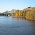Is the circuit able to detect current direction? If not, can it be modified to detect it?

1.The chip datasheet clearly indicates that it is bidirectional, which is useful when the load is a battery. I haven't tried this condition, but I can soon test it.

3.I can detect the current direction

1.Sorry, I realized that I had made a mistake in the diagram in figure 6, now I have corrected it.

4.Impressive and powerful suggestion by the author of this blog are really helpful to me. click read

5.Very good article . Btw - The resolution of the shunt is 2.5uV (micro Volt) and not 2.5mV as stated.

6.In my post I wrote several times that the resolution of the shunt voltage is 2.5 uV, I don't know where you read 2.5 mV. Let me know, thanks.

7.Your blog is a great source of learning for me. I encourage you to please keep posting such projects.